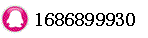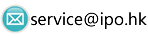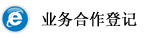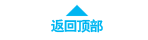# generalized method of moment时间：2018-04-25 22:35:13     浏览：106    评论：0

Generalized method of moments
GMM may also mean Gaussian mixture model.
The generalized method of moments is a very general statistical method for obtaining estimates of parameters of statistical models. It is a generalization, developed by Lars Peter Hansen, of the method of moments.
The term GMM is very popular among econometricians but is hardly used at all outside of economics, where the slightly more general term estimating equations is preferred. The method is also closely related to the classical theory of minimum chi-square estimation.
Description
The idea of the generalized method of moments is to use moment conditions that can be found from the problem with little effort. We assume that the data are a stochastic process In mathematical language, we start out with a function f that depends both on the parameter and a single observation and that has mean zero for the true value of the parameter, θ = θ0, i.e.
图（1)
To turn this function into a parameter estimate, we minimize the associated chi-square statistic
图
where superscript T is used for transpose, and A is a matrix. A may be known a priori or estimated from the sample.
由于技术原因图1无法上传，
详见
http://en.wikipedia.org/wiki/Generalized_method_of_moments

0相关评论联系我们